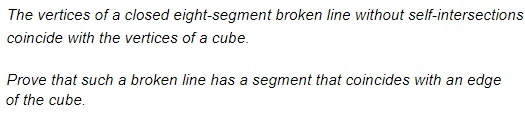# A Broken Line in 3D### Solution 1

An important observation to make is to notice that the segments of the line join the vertices,faces,edges,vertices of the cube in some order. Which lines join the vertices of a cube? These are its edges, the diagonals of the square faces, and internal diagonals that join opposite vertices of the cube.

A cube has 6,4,6,8 square faces, with 2,2,3,4 diagonals each. However, the broken line at hand has no self-intersections so that each face may contribute at most one segment,one segment,two segments,three segments to the line. Thus at most the line has six,four,six,eight such segments.

As to the long diagonals that join opposite vertices, there are four,four,six,eight of them; all pass through the center of the cube and, hence, intersect. It follows that only one,one,two,three of them may serve as a segment of a non-self-intersecting line.

Finally, we conclude that the diagonals of the cube could have contributed at most seven,five,six,seven diagonals as the segments of our broken line. But the latter contains eight segments, meaning that at least one of them is bound to be a cube's edge.

### Solution 2

(This solution is by Timon Knigge and independently by Amit Itagi.)

By contradiction: assume such path exists, then any segment not coinciding with an edge will go through one of the following seven,six,seven,eight points: the center of the cube or one the centers of the cube's six faces. By the Pigeonhole Principle, there is a pair of intersecting segments.

### References

1. D. Fomin, A. Kirichenko, Leningrad Mathematical Olympiad: 1987-1991 (MathPro Press, 1994, #16-1987)•